# Maharashtra Board Practice Set 13 Class 7 Maths Solutions Chapter 3 HCF and LCM

Balbharti Maharashtra State Board Class 7 Maths Solutions covers the 7th Std Maths Practice Set 13 Answers Solutions Chapter 3 HCF and LCM.

## HCF and LCM Class 7 Practice Set 13 Answers Solutions Chapter 3

Question 1.
Find the LCM:
i. 12, 15
ii. 6, 8, 10
iii. 18, 32
iv. 10, 15, 20
v. 45, 86
vi. 15, 30, 90
vii. 105, 195
viii. 12,15,45
ix. 63,81
x. 18, 36, 27
Solution:
i. 12, 15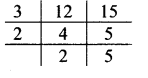∴ LCM of 12 and 15 = 3 x 2 x 2 x 5
= 60

ii. 6, 8, 10∴ LCM of 6, 8 and 10 = 2 x 2 x 3 x 2 x 5
= 120

iii. 18, 32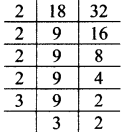∴ LCM of 18 and 32 = 2 x 2 x 2 x 2 x 3 x 3 x 2
= 288

iv. 10, 15, 20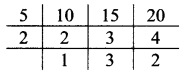∴ LCM of 10, 15 and 20 = 5 x 2 x 3 x 2
= 60

v. 45, 86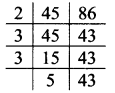∴ LCM of 45 and 86 = 2 x 3 x 3 x 5 x 43
= 3870

vi. 15, 30, 90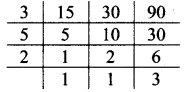∴ LCM of 15,30 and 90 = 3 x 5 x 2 x 3
= 90

vii. 105, 195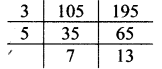∴ LCM of 105 and 195 = 5 x 3 x 7 x 13
= 1365

viii. 12, 15, 45∴ LCM of 12, 15 and 45 = 3 x 3 x 2 x 5 x 2
= 180

ix. 63, 81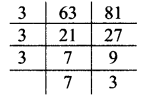∴ LCM of 63 and 81 = 3 x 3 x 3 x 7 x 3
= 567

x. 18, 36, 27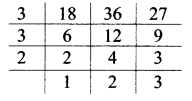∴ LCM of 18, 36 and 27 = 3 x 3 x 2 x 2 x 3
= 108

Question 2.
Find the HCF and LCM of the numbers given below. Verify that their product is equal to the product of the given numbers:
i. 32, 37
ii. 46, 51
iii. 15, 60
iv. 18, 63
v. 78, 104
Solution:
i. 32 = 2 x 16
= 2 x 2 x 8
= 2 x 2 x 2 x 4
= 2 x 2 x 2 x 2 x 2 x 1
37 = 37 x 1
∴ HCF of 32 and 37 =1
LCM of 32 and 37 = 2 x 2 x 2 x 2 x 2 x 37
= 1184
HCF x LCM = 1 x 1184
= 1184
Product of the given numbers = 32 x 37
= 1184
∴ HCF x LCM = Product of the given numbers.

ii. 46 = 2 x 23 x 1
51 = 3 x 17 x 1
∴ HCF of 46 and 51 = 1
LCM of 46 and 51 = 2 x 23 x 3 x 17
= 2346
HCF x LCM = 1 x 2346
= 2346
Product of the given numbers = 46 x 51
= 2346
∴ HCF x LCM = Product of the given numbers.

iii. 15 = 3 x 5
60 = 2 x 30
= 2 x 2 x 15
= 2 x 2 x 3 x 5
∴ HCF of 15 and 60 = 3 x 5
= 15
LCM of 15 and 60 = 3 x 5 x 2 x 2
= 60
HCF x LCM = 15 x 60
= 900
Product of the given numbers = 15 x 60
= 900
∴ HCF x LCM = Product of the given numbers.

iv. 18 = 2 x 9
= 2 x 3 x 3
63 = 3 x 21
= 3 x 3 x 7
∴ HCF of 18 and 63 = 3 x 3
= 9
LCM of 18 and 63 = 3 x 3 x 2 x 7
= 126
HCF x LCM = 9 x 126
= 1134
Product of the given numbers = 18 x 63
= 1134
∴ HCF x LCM = Product of the given numbers.

v. 78 = 2 x 39
= 2 x 3 x 13
104 = 2 x 52
= 2 x 2 x 26
= 2 x 2 x 2 x 13
∴ HCF of 78 and 104 = 2 x 13
= 26
LCM of 78 and 104 = 2 x 13 x 3 x 2 x 2
= 312
HCF x LCM = 26 x 312
= 8112
Product of the given numbers = 78 x 104
= 8112
∴ HCF x LCM = Product of the given numbers.

Maharashtra Board Class 7 Maths Chapter 3 HCF and LCM Practice Set 13 Intext Questions and Activities

Question 1.
Write the tables of the given numbers and find their LCM. (Textbook pg. no. 19)
i. 6, 7
ii. 8, 12
iii. 5, 6, 15
Solution:
i. Multiples of 6 : 6, 12, 18, 24, 30, 36, 42
Multiples of 7 : 7, 14, 21, 28, 35, 42, 49
∴ LCM of 6 and 7 = 42

ii. Multiples of 8 : 8, 16, 24, 32, 40
Multiples of 12 : 12, 24, 36, 48
∴ LCM of 8 and 12 = 24

iii. Multiples of 5 : 5, 10, 15, 20, 25, 30, 35
Multiples of 6 : 6, 12, 18, 24, 30, 36
Multiples of 15 : 15, 30, 45, 60
∴ LCM of 5,6 and 15 = 30

Scroll to Top